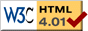# Double Spring

previous next
• Sim
• Graph
• Time Graph
• Multi Graph

This simulation shows two springs and masses connected to a wall. The graphs produced are called Lissajous curves and are generated by simple sine and cosine functions.

You can change parameters in the simulation such as mass or spring stiffness. You can drag either mass with your mouse to set the starting position.

The math behind the simulation is shown below. Also available are: open source code, documentation and a simple-compiled version which is more customizable.

## Physics and Equations of Motion

The two springs act independently, so it is easy to figure out what are the forces acting on the two blocks. Label the springs and blocks as follows:

wall - spring1 - block1 - spring2 - block2

We'll assume the origin is at the connection of the spring to the wall. Define the following variables (subscripts refer to block 1 or block 2):

• x1, x2 = position (left edge) of blocks
• v1, v2 = velocity of blocks
• F1, F2 = force experienced by blocks
• L1, L2 = how much spring is stretched

And define the following constants:

• m1, m2 = mass of blocks
• w1, w2 = width of blocks
• k1, k2 = spring constants
• R1, R2 = rest length of springs

The springs exert force based on their amount of stretch according to

F = −k × stretch

The forces on the blocks are therefore

F1 = −k1 L1 + k2 L2
F2 = −k2 L2

The stretch of the spring is calculated based on the position of the blocks.

L1 = x1R1
L2 = x2x1w1R2

Now using Newton's law F = m a and the definition of acceleration as a = x'' we can write two second order differential equations. These are the equations of motion for the double spring.

m1 x1'' = −k1 (x1R1) + k2 (x2x1w1R2)
m2 x2'' = −k2 (x2x1w1R2)

## Numerical Solution

It is easy to convert the above second order equations to a set of first order equations. We define variables for the velocities v1, v2 . Then there are four variables x1, x2, v1, v2 and a first-order differential equation for each:

x1' = v1
x2' = v2
v1' = −(k1m1) (x1R1) + (k2m1) (x2x1w1R2)
v2' = −(k2m2) (x2x1w1R2)

This is the form that we need in order to use the Runge-Kutta method for numerically solving the differential equation.

This web page was first published April 2001.

previous next